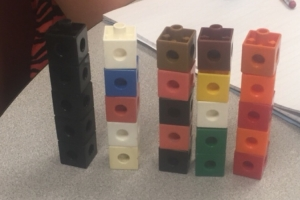# Stay Tuned For “Mean”

As a follow-up to the lesson I posted yesterday (See:  Diagnostic:  Mode, Median & Mean) we gave this question to the students:

The number of goals scored by a hockey team are as follows:

Game 1:  4           Game 2:  2           Game 3:  6           Game 4:  4           Game 5:  9

1.  What is the mode number of goals scored?
2. What is the median number of goals scored?
3. If the team had scored the same number of goals each game, how many would that be each time?Photo 1: Creating the bar graph using snap cubes.

After reading the task to the whole group we asked the students to build a concrete graph using snap cubes to represent the games and the number of goals.  (Photo 1)

For Question 1, they could compare bars and see if there were any of equal length to figure out the mode.

For Question 2, the bars could easily be arranged from shortest to longest to find the median

For Question 3, we asked the students to use only the snap cubes in their graph to figure out the answer.  Students got busy moving cubes until they had 5 columns of equal length. (Photo 2)

The students discovered that if we took the number of goals 4, 2, 6, 4 and 9 for a total of 25 and shared them equally among the 5 games, there were 5 each.Photo 2: Balancing the bars to make equal groups.

Of course, as you can see, we are having the students construct their understanding of “mean” with a task like this.  So far, we have not told them this, but will do so after more guided lessons involving the balancing of sets of numbers using the snap cubes.

Working with the snap cubes like this will bring meaning to the formula:  “To calculate the mean, add up all of the numbers and divide by that many numbers:  e.g., 4+2+6+4+9=25      25÷5=5

Hopefully in the future, when students are wondering what mode, median, and mean are they will remember the “actions” they performed with the snap cubes.

Mode:  “Compared” bars to find ones of equal length.

Median:  “Ordered” the bars from least to greatest to find the middle.

Mean:  “Balanced” the bars to create all equal lengths/

Of course, the snap cubes will only work with numbers that can be divided equally, but we will let students discover and deal with that after there is a firm understanding of the concept.